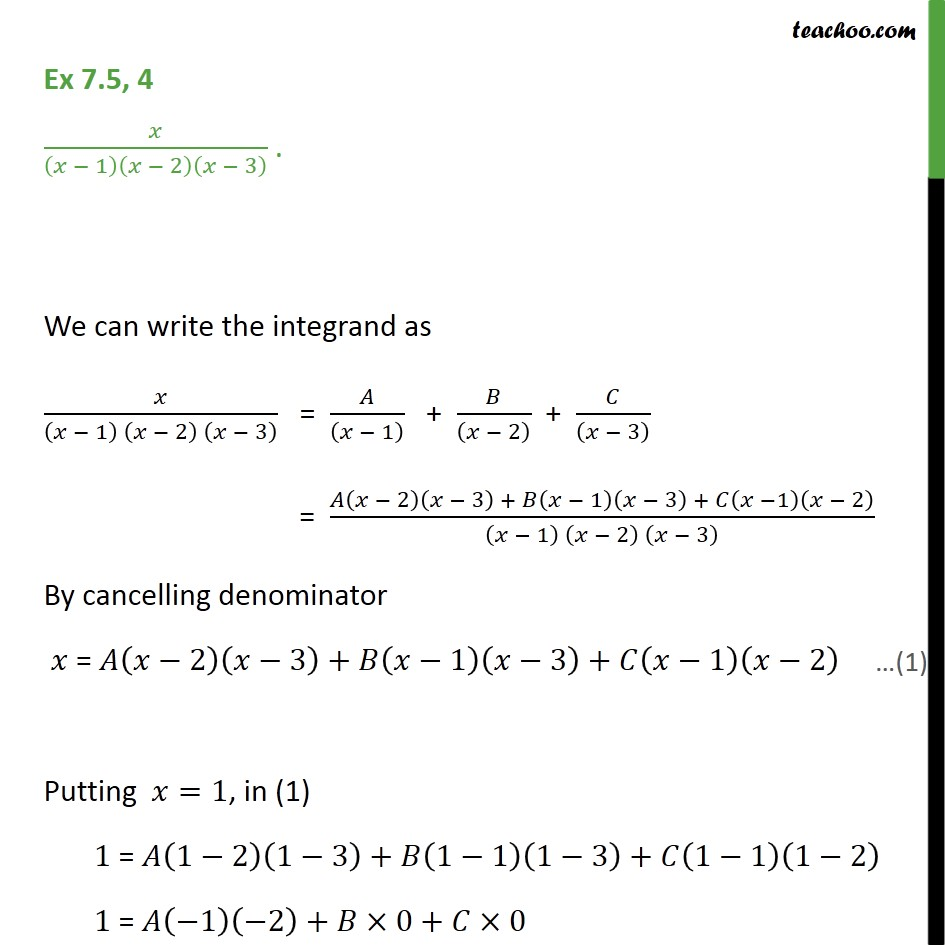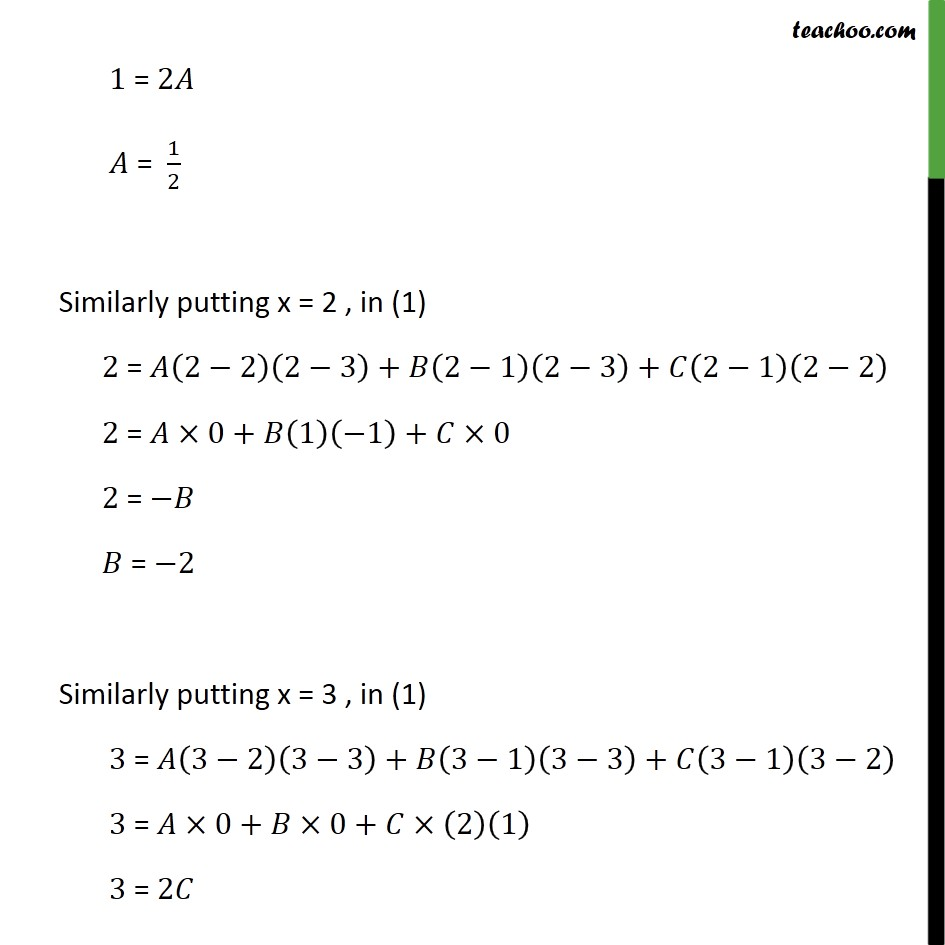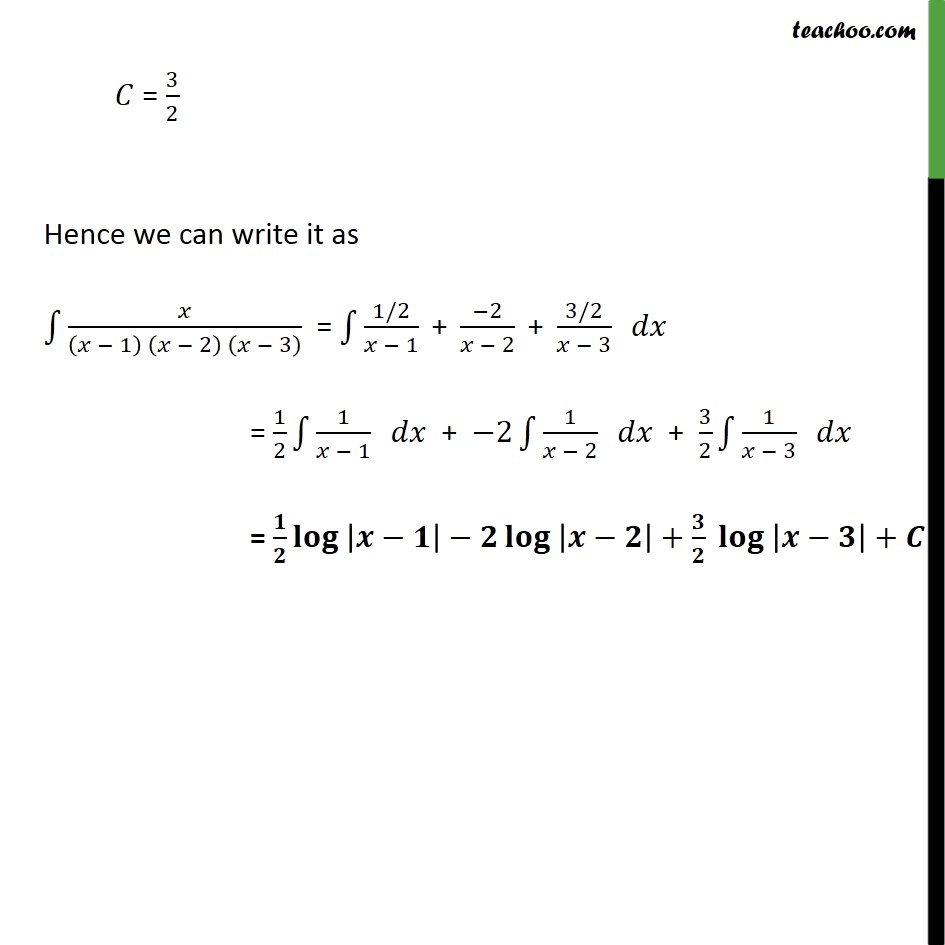Ex 7.5

Chapter 7 Class 12 Integrals
Serial order wiseLearn in your speed, with individual attention - Teachoo Maths 1-on-1 Class

### Transcript

Ex 7.5, 4 𝑥/(𝑥 − 1)(𝑥 − 2)(𝑥 − 3) . We can write the integrand as 𝑥/((𝑥 − 1) (𝑥 − 2) (𝑥 − 3) ) = 𝐴/((𝑥 − 1) ) + 𝐵/((𝑥 − 2) ) + 𝐶/((𝑥 − 3) ) = (𝐴(𝑥 − 2)(𝑥 − 3) + 𝐵(𝑥 − 1)(𝑥 − 3) + 𝐶(𝑥 −1)(𝑥 − 2))/((𝑥 − 1) (𝑥 − 2) (𝑥 − 3) ) By cancelling denominator 𝑥 = 𝐴(𝑥−2)(𝑥−3)+𝐵(𝑥−1)(𝑥−3)+𝐶(𝑥−1)(𝑥−2) Putting 𝑥=1, in (1) 1 = 𝐴(1−2)(1−3)+𝐵(1−1)(1−3)+𝐶(1−1)(1−2) 1 = 𝐴(−1)(−2)+𝐵×0+𝐶×0 1 = 2𝐴